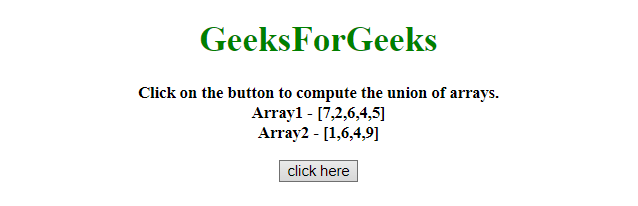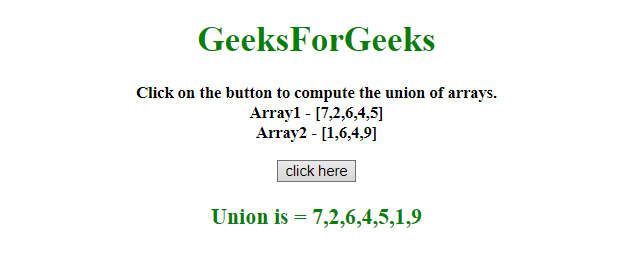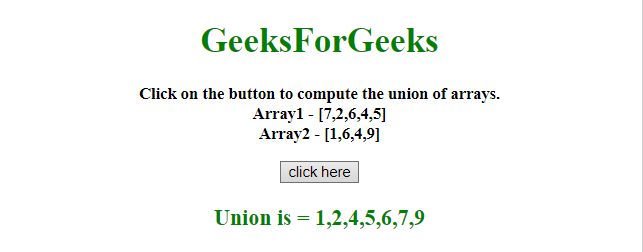# How to compute union of JavaScript arrays ?

Given two arrays containing array elements and the task is to compute the union of both arrays with the help of JavaScript. There are two methods to solve this problem which are discussed below:

Approach 1:

• Declare both array named as A and B.
• Use spread operator to concate both array and store it into set.
• Since, set removes the duplicate elements.
• After removinf the duplicate elements, it will display the union of array element.

Example: This example implements the above approach.

 `  ` `<``html``>  ` ` `  `<``head``>  ` `    ``<``title``>  ` `        ``How to compute union of ` `        ``JavaScript arrays ? ` `    `` ` ` ` ` `  `<``body` `style` `= ``"text-align:center;"``>  ` `     `  `    ``<``h1` `style` `= ``"color:green;"` `id` `= ``"h1"``>  ` `        ``GeeksForGeeks  ` `    `` ` `     `  `    ``<``p` `id` `= ``"GFG_UP"` `style` `= ` `        ``"font-size: 15px; font-weight: bold;"``> ` `    `` ` `     `  `    ``<``button` `onclick` `= ``"GFG_Fun()"``> ` `        ``click here ` `    `` ` `     `  `    ``<``p` `id` `= ``"GFG_DOWN"` `style` `=  ` `        ``"color:green; font-size: 20px; font-weight: bold;"``> ` `    `` ` `     `  `    ``<``script``>  ` `        ``var up = document.getElementById('GFG_UP'); ` `        ``var down = document.getElementById('GFG_DOWN'); ` `        ``var A = [7, 2, 6, 4, 5]; ` `        ``var B = [1, 6, 4, 9]; ` `         `  `        ``up.innerHTML = "Click on the button to compute" ` `                    ``+ " the union of arrays.<``br``>"  ` `                    ``+ "Array1 - [" + A + "]<``br``>Array2 - ["  ` `                    ``+ B + "]"; ` `         `  `        ``function GFG_Fun() { ` `            ``var union = [...new Set([...A, ...B])]; ` `            ``down.innerHTML = "Union is = " + union; ` `        ``} ` `    ``  ` `    ``  ` ` `

Output:

• Before clicking on the button:• After clicking on the button:Approach 2:

• Here, all elements are stored in a JavaScript object.
• Storing elements in JavaScript Object is going to remove the duplicate elements.
• At the end, push all element in a JavaScript array.

Example: This example implements the above approach.

 `  ` `<``html``>  ` ` `  `<``head``>  ` `    ``<``title``>  ` `        ``How to compute union of ` `        ``JavaScript arrays ? ` `    `` ` ` ` ` `  `<``body` `style` `= ``"text-align:center;"``>  ` `     `  `    ``<``h1` `style` `= ``"color:green;"` `id` `= ``"h1"``>  ` `        ``GeeksForGeeks  ` `    `` ` `     `  `    ``<``p` `id` `= ``"GFG_UP"` `style` `= ` `        ``"font-size: 15px; font-weight: bold;"``> ` `    `` ` `     `  `    ``<``button` `onclick` `= ``"GFG_Fun()"``> ` `        ``click here ` `    `` ` ` `  `    ``<``p` `id` `= ``"GFG_DOWN"` `style` `= ` `        ``"color:green; font-size: 20px; font-weight: bold;"``> ` `    `` ` `     `  `    ``<``script``>  ` `        ``var up = document.getElementById('GFG_UP'); ` `        ``var down = document.getElementById('GFG_DOWN'); ` `        ``var A = [7, 2, 6, 4, 5]; ` `        ``var B = [1, 6, 4, 9]; ` `         `  `        ``up.innerHTML = "Click on the button to compute" ` `            ``+ " the union of arrays.<``br``>" + "Array1 - [" ` `            ``+ A + "]<``br``>Array2 - [" + B + "]"; ` `         `  `        ``function computeUnion(a, b) { ` `            ``var object = {}; ` `             `  `            ``for (var i = a.length-1; i >= 0; -- i) ` `                ``object[a[i]] = a[i]; ` `             `  `            ``for (var i = b.length-1; i >= 0; -- i) ` `                ``object[b[i]] = b[i]; ` `             `  `            ``var ret = [] ` `             `  `            ``for (var i in object) { ` `                ``if (object.hasOwnProperty(i)) ` `                ``ret.push(object[i]); ` `            ``} ` `             `  `            ``return ret; ` `        ``} ` `         `  `        ``function GFG_Fun() { ` `            ``down.innerHTML = "Union is = "  ` `                    ``+ computeUnion(A, B); ` `        ``} ` `    ``  ` `  ` ` `  ` `

Output:

• Before clicking on the button:• After clicking on the button:My Personal Notes arrow_drop_upCheck out this Author's contributed articles.

If you like GeeksforGeeks and would like to contribute, you can also write an article using contribute.geeksforgeeks.org or mail your article to contribute@geeksforgeeks.org. See your article appearing on the GeeksforGeeks main page and help other Geeks.

Please Improve this article if you find anything incorrect by clicking on the "Improve Article" button below.